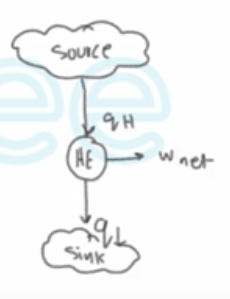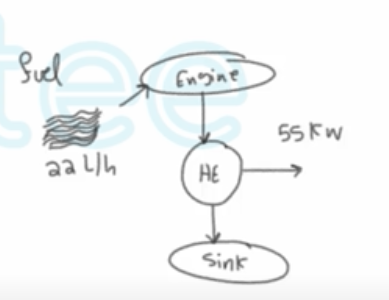Need Help?

Subscribe to Thermodynamics

###### \${selected_topic_name}
• Notes

$\begin{array}{l}{\text { A heat engine that pumps water out of an underground mine accepts } 700 \mathrm{kJ} \text { of heat }} \\ {\text { and produces } 250 \mathrm{kJ} \text { of work. How much heat does it reject, in } \mathrm{kJ} \text { ? }}\end{array}$$\eta_{t h}=\frac{w_{n e t}}{q_{H}} \rightarrow w_{n e t}=\eta_{t h} * q_{H}$

$\rightarrow q_{H}-q_{L}=\eta_{t h}* q_H$

$\rightarrow q_{H}=\frac{q_{L}}{1-\eta_{t h}}=\frac{1000}{1-0.4}$

$∴ q_{H}=1667 \mathrm{kJ} / \mathrm{kg}$

$\begin{array}{l}{\text { A steam power plant with a power output of } 150 \mathrm{MW} \text { consumes coal at }} \\ {\text { a rate of } 60 \text { tonsh. If the heating value of the coal is } 30,000 \mathrm{kJ} / \mathrm{kg} \text { , }} \\ {\text { determine the overall efficiency of this plant. }}\end{array}$$\dot m_{\rho vel}=\rho \dot{V}=0.8 * 22 \ \mathrm{l/h}$

$=17.6 \mathrm{kg} / \mathrm{h}$

$\dot{Q}_{H}=\dot{m} q_{H}$

$=17.6 * 44000=774400 \mathrm{kJ} / \mathrm{h}$

$=215.1 \mathrm{kw}$

$\rightarrow \eta_{th}=\frac{\dot{W}_{net}}{\dot Q_{H}}=\frac{55kw}{215.1kw}*100 \%$

$=25.6 \%$